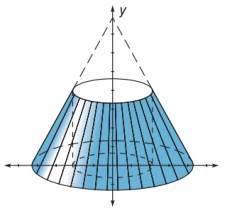Chapter 10.1, Problem 42E### Elementary Geometry for College St...

6th Edition
Daniel C. Alexander + 1 other
ISBN: 9781285195698

#### Solutions

Chapter
Section### Elementary Geometry for College St...

6th Edition
Daniel C. Alexander + 1 other
ISBN: 9781285195698
Textbook Problem
1 views

# Find the volume of the solid formed when the triangular region having vertices at 2, 0 , 4, 0 , and 2, 4 is rotated about the y -axis.To determine

To show:

The volume of solid when the triangular region having vertices 2, 0, 4, 0 and 2, 4 is rotated about the y-axis.

Explanation

A triangle is drawn with the following vertices 2, 0, 4, 0 and 2, 4.

Now, the triangle is rotated about the y-axis.

The resultant will be a cone.

Now, we have to find the volume of the cone.

Let h be the height of the cone.

Let r be the radius of the cone.

From the diagram,

Height is the distance between the points 2, 4 and 2, 0.

x1,y1=2, 4

x2,y2=2, 0

Formula for distance between two points

d=x2-x12+y2-y12

Substituting the x and y co-ordinates

d=2-22+0-42

On solving this,

d=02+-42

d=42

d=16

d=4

Hence, height is 4 units.

Radius is the distance between the points 2, 0 and 4, 0

### Still sussing out bartleby?

Check out a sample textbook solution.

See a sample solution

#### The Solution to Your Study Problems

Bartleby provides explanations to thousands of textbook problems written by our experts, many with advanced degrees!

Get Started

#### Convert the expressions in Exercises 8596 radical form. 45x3/2

Finite Mathematics and Applied Calculus (MindTap Course List)

#### Evaluate the integral, if it exists. 04x1dx

Single Variable Calculus: Early Transcendentals

#### The graph of is: a) b) c) d)

Study Guide for Stewart's Single Variable Calculus: Early Transcendentals, 8th